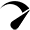# Lithium (LiFePO4) Battery Charge Time Calculator & Formula

Use our lithium battery charge time calculator to find out how long it will take to charge a lithium battery with solar panels or with a battery charger.

Two columns
Vertical
Horizontal

## Battery runtime(Hours)

{{ field.label }} {{ field.converted }} {{ field.extraView }}
{{ option.label }} {{ option.converted }}
{{ item.label }} {{ item.data.converted }}

Issued on: {{ \$store.getters.getIssuedOn }}
Payment method: {{ \$store.getters.getPaymentType }}
{{ item.label }}: {{ item.converted }}

### Calculator Assumptions

• Lithium battery charge efficiency – 95%
• Charge controller efficiency – PWM: 80%, MPPT: 98%
• Solar panel efficiency – 80%

### How To Use This Calculator? (Example)

1. Enter the battery capacity in amp-hours (Ah). Let’s say you have a 100ah lithium battery (enter 100).
2. Enter the battery volts. Is this a 12, 24, or 48-volt battery? Let’s say you have a 12v battery (Enter 12).
3. Enter the battery depth of discharge (DoD): Battery Depth of discharge refers to the percentage of a battery that has been discharged relative to the overall capacity of the battery. For example, if your battery is discharged at 80%, enter 80.
4. Enter the solar panel size in watts. If you have multiple solar panels connected together, add up their rated wattage and enter the number (2 x 100W = 200W).
5. Select the charge controller type. Are you using a PWM or an MPPT charge controller? Choose accordingly.

## How Do You Calculate Lithium-Ion Battery Charging Time?

Here are the methods to calculate lithium (LiFePO4) battery charge time with solar and battery chargers.

Formula: charge time = (battery capacity Wh × depth of discharge) ÷ (solar panel size × Charge controller efficiency × charge efficiency × 80%)

Battery depth of discharge (DoD): Battery Depth of discharge refers to the percentage of a battery that has been discharged relative to the overall capacity of the battery.

Lithium battery charge efficiency: 90 – 95% `(Source)`

Note: In the real world, the battery charge efficiency rate will not be fixed and will depend on a number of factors. Like the battery charge rate (the faster the charge rate is the less efficient it is) and the battery depth state of charge (SOC).

Charge controller efficiency – PWM: 80%, MPPT: 98%

Battery capacity in watt-hours (Wh) Battery Ah × Battery volts

#### Example

Let’s suppose

• Battery capacity: 12v 100Ah lithium battery (100 × 12 = 1200Wh)
• Solar panel size (W): 200 watt
• Charge controller type: MPPT
• DoD: 80%
``Charge time = (1200 × 80%) ÷ (200 × 98%  × 95% × 80%)``
``Charge time: (960) ÷ (148.9) = 6.4 hours``

### 2: Lithium Battery Charge Time Using Battery Charger

Formula: charge time = (battery capacity × depth of discharge) ÷ (charge current × charge efficiency)

Note: Enter the battery capacity in Ah or mAh if the charger current output is mentioned in amps (A) or milliamps (mA). However, if the output value of the charger is mentioned in watts (W) or watt-hours (Wh), enter the battery capacity in watt-hours (Wh).

#### Example

Let’s suppose

• Battery Capacity: 100Ah
• Battery DoD: 100%
• Charge current: 10A
``Charge time: (100 × 100%) ÷ (10 × 95%)``
``Charge time: (100) ÷ (9.5) = 10.5 hours``

100Ah lithium battery will take about 10.5 hours to get fully charged from 100% depth of discharge (0% SoC) using a 10A charger.

## How Long To Charge A Lithium (LiFePO4) Battery?

Calculating the battery’s exact charge time is not an easy task. However, you can use our above lithium battery charge time calculators or formulas to get an estimated battery charge time.

Scroll to TopOptimized by Seraphinite Accelerator
Turns on site high speed to be attractive for people and search engines.